• Save

# Figural Classification Questions for Competitive Exams

3 months ago 879 Views
NEW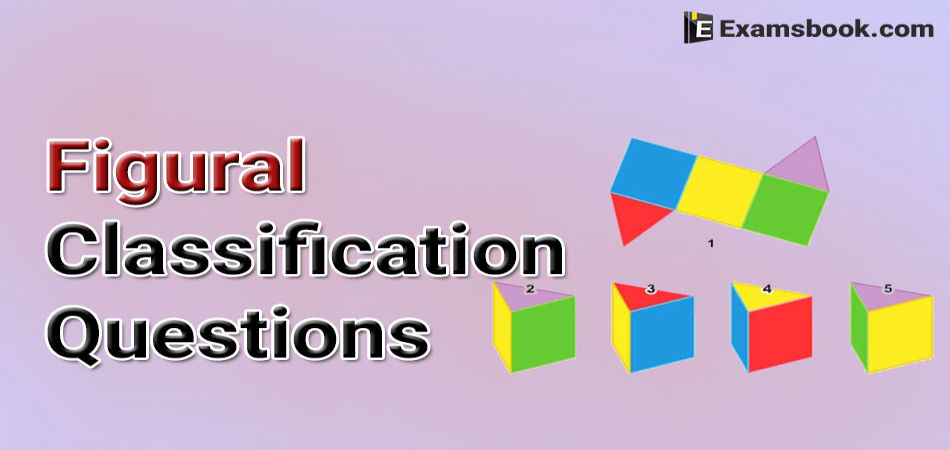Some students are get puzzled to solve figural questions in the competitive exams because they can't understand this topic properly. So, here I am sharing figural classification questions with their answers for your practice.

You can easily understand this topic by these questions and definition. So,  continue your preparation with these questions and obtain good marks in the competitive exams.

As well as, you can practice Number Classification Reasoning Questions for better preparation.

## Figural Classification Questions and Answers

In such type of question, a group four figures is given in them three belongs to a common group with their properties and one belongs to another group. Candidates are required to choose that one figure, which does not belong to the common group. This type of approach of finding the odd figure known as figural classification.

Example.1.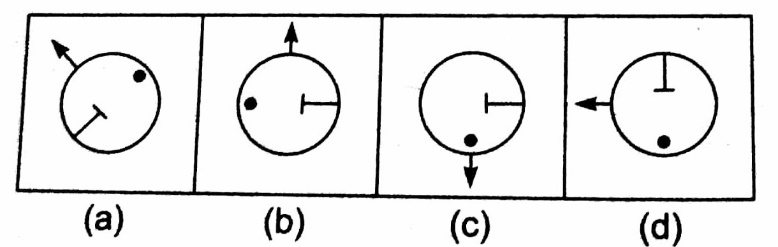Ans. C

In figure, (c) the dot point ‘●’ is to the left of ‘T’, while in another dot point ‘●’ appears to the opposite of ‘T’.

Q.1.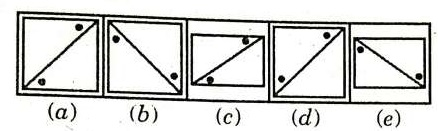Ans .   D

The two dots lie on the same side of the diagonal only in fig. (d)

Q.2.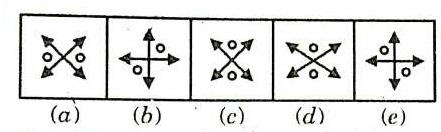Ans .   D

In all other figures, the two double sided arrows intersect each other at right angle.

Q.3.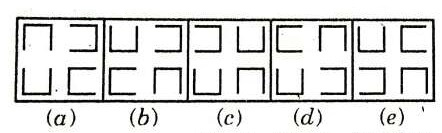Ans .   C

only in fig. (c), two out of the four elements are opening in the same direction

Q.4.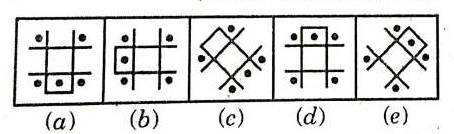Ans .   C

All other figures can be rotated into each other.

Q.5.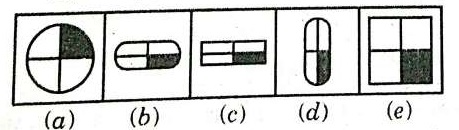Ans .   A

In all other figures, the lower-right quarter portion is shaded.

Q.6.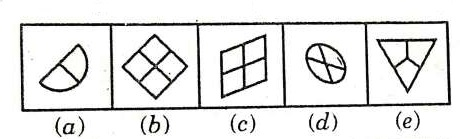Ans .   D

All other figures are divided into equal parts.

Q.7.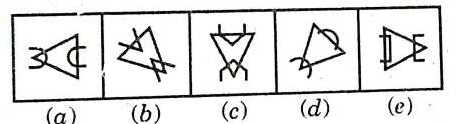Ans .   D

Only in fig. (d), the two similar elements intersection the triangle opens up in the same direction.

Q.8.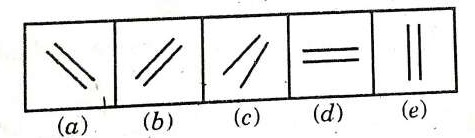Ans .   C

In all other figures, the tow line segments are parallel to each other.

Q.9.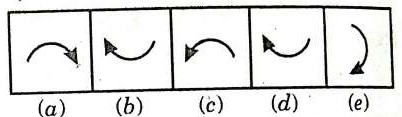Ans .   A

All other figures can be rotated into each other.

Q.10.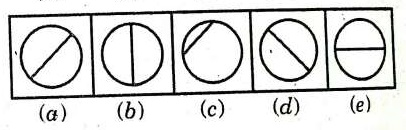Ans .   C

In fig (c), the arrow indicates an ACW motion while in each of the other figures the arrow indicates a CW motion.